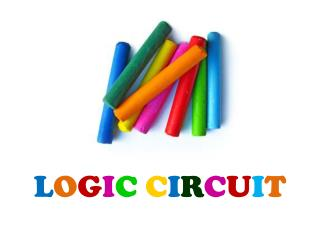Download PresentationL O G I C C I R C U I TL O G I C C I R C U I T - PowerPoint PPT Presentation

Download PresentationL O G I C C I R C U I T
An Image/Link below is provided (as is) to download presentation

Download Policy: Content on the Website is provided to you AS IS for your information and personal use and may not be sold / licensed / shared on other websites without getting consent from its author. While downloading, if for some reason you are not able to download a presentation, the publisher may have deleted the file from their server.

- - - - - - - - - - - - - - - - - - - - - - - - - - - E N D - - - - - - - - - - - - - - - - - - - - - - - - - - -
Presentation Transcript

1. LOGICCIRCUIT

2. Goal • To understand how digital a computer can work, at the lowest level. • To understand what is possible and the limitations of what is possible for a digital computer.

3. Logic Gates • Digital circuits are hardware components that manipulate binary information. • Logic gates are implemented using transistors and integrated circuits. • Each basic circuit is referred to as a logic gate.

4. Logic Gates • All basic logic gates have the ability to accept either one or two input signals (depending upon the type of gate) and generate one output signal.

5. Logic Gates Symbols Inputs and outputs • Gates have two or more inputs, except a NOT gate which has only one input. All gates have only one output. Usually the letters A, B, C and so on are used to label inputs, and Q is used to label the output. On this page the inputs are shown on the left and the output on the right. The inverting circle (o) • Some gate symbols have a circle on their output which means that their function includes inverting of the output. It is equivalent to feeding the output through a NOT gate. For example the NAND (Not AND) gate symbol shown on the right is the same as an AND gate symbol but with the addition of an inverting circle on the output.

6. Basic logic gates • NOT • AND • OR • NAND • NOR

7. Truth Table • A truth table is a good way to show the function of a logic gate. • It shows the output states for every possible combination of input states. • The symbols 0 (false) and 1 (true) are usually used in truth tables.

8. Logic Gate: NOT • The NOT gate is also known as an inverter, simply because it changes the input to its opposite (inverts it).  • The NOT gate accepts only one input and the output is the opposite of the input.  • A common way of using the NOT gate is to simply attach the circle to the front of another gate.  This simplifies the circuit drawing and simply says: "Invert the output from this gate."

9. Logic Gate: AND • The AND gate requires two inputs and has one output.  • The AND gate only produces an output of 1 when BOTH the inputs are a 1, otherwise the output is 0.

10. Logic Gate: OR • The AND gate requires two inputs and has one output.  • The AND gate only produces an output of 1 when BOTH the inputs are a 1, otherwise the output is 0.

11. Logic Gate: NAND • This is an AND gate with the output inverted, as shown by the 'o' on the output. • The output is true if input A AND input B are NOT both true: Q = NOT (A AND B) • A NAND gate can have two or more inputs, its output is true if NOT all inputs are true.

12. Logic Gate: NOR • This is an OR gate with the output inverted, as shown by the 'o' on the output. • The output Q is true if NOT inputs A OR B are true: Q = NOT (A OR B) • A NOR gate can have two or more inputs, its output is true if no inputs are true.

13. Logic Circuit Design

14. Logic Circuit DesignExample 1 Find the Boolean expressions output of the following circuit: Answer: (x+y)y

15. ___ _ _ Logic Circuit DesignExample 2 Find the Boolean expressions output of the following circuit: Answer: xy

16. x+y x Logic Circuit DesignExample 3 Draw the circuits for the following Boolean algebraic expressions: x+y __

17. (x+y)x (x+y)x x+y Logic Circuit DesignExample 4 Draw the circuits for the following Boolean algebraic expressions: x+y

18. A AND AND B Y OR NOT C A B C Y 0 0 0 0 0 1 0 1 0 0 1 1 1 0 0 1 0 1 1 1 0 1 1 1 Circuit-to-Truth Table Example 2# ofInputs = # of Combinations 2 3 = 8

19. A A B A AND AND B Y = A B + A C OR } NOT } C A C A B C Y 0 0 0 0 0 0 0 1 1 0 1 0 0 0 1 1 1 1 0 0 0 1 0 1 0 1 1 0 1 1 1 1 1 Circuit-to-Truth Table Example